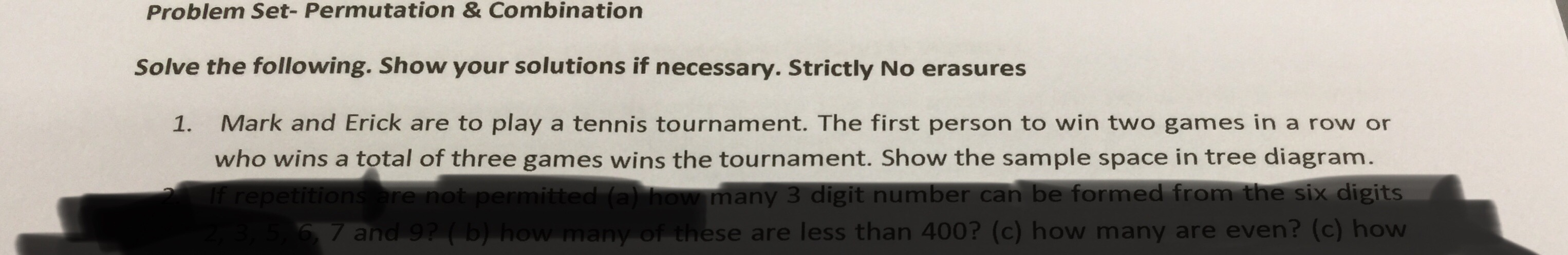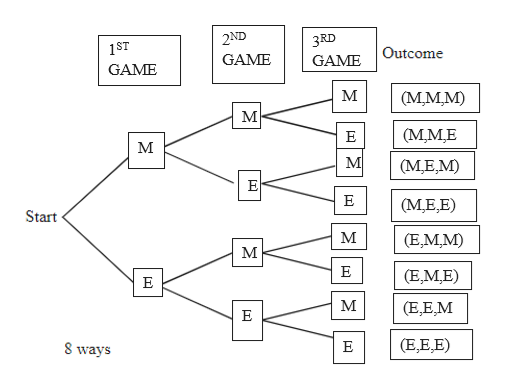Problem Set- Permutation & CombinationSolve the following. Show your solutions if necessary. Strictly No erasures1.Mark and Erick are to play a tennis tournament. The first person to win two games in a row orwho wins a total of three games wins the tournament. Show the sample space in tree diagram.if repetitions are not permitted (a) how many 3 digit number can be formed from the six digitsof these are less than 400? (c) how7 and 9? (b) how ma6,are even? (c) how

Questionhelp_outlineImage TranscriptioncloseProblem Set- Permutation & Combination Solve the following. Show your solutions if necessary. Strictly No erasures 1. Mark and Erick are to play a tennis tournament. The first person to win two games in a row or who wins a total of three games wins the tournament. Show the sample space in tree diagram. if repetitions are not permitted (a) how many 3 digit number can be formed from the six digits of these are less than 400? (c) how 7 and 9? (b) how ma 6, are even? (c) how fullscreen
Step 1

There are two ways both Mark and Erick can win the tournament that is either they win  two game or all three of them and the  possible combined choices are called as outcomes.

Step 2

The outcomes can be shown through a tree diagram.

Here M denote as Mark and E denote as...help_outlineImage Transcriptionclose2ND 3RD 1ST Outcome GAME GAME GAME (М.мM) (МMЕ Е м М (М.Е,M) E (М.Е.E) Start м (E,MM) М E (E,ME) Е м (E,E,M E (E,E,E) 8 ways Е [T] fullscreen

Want to see the full answer?

See Solution

Want to see this answer and more?

Our solutions are written by experts, many with advanced degrees, and available 24/7

See Solution
Tagged in

Permutations and Combinations